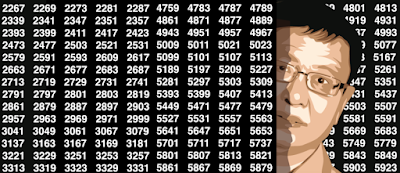## Wednesday, January 31, 2018

### Four Interesting Math Problems

The level in this article is for college students familiar with calculus, This material will be also of interest to college professors looking for new material to teach, or for original exam questions, as well as for business data scientists with some spare time, interested in refreshing their math skills. The problems cover real analysis, mathematical algorithms and numerical precision, correct visualizations, as well as geometry. The third problem is the most interesting one in my opinion, and could become a subject of active mathematical research with one new great, unsolved conjecture being proposed, of a probabilistic nature. The last problem has many applications in engineering science.
1. The Simplest Function Defined by an Infinite Product
• Exercise
2. Surprising Series for Powers of Number 2
3. From Continuous Fractions to Nested Square Roots and More
• Algorithm to compute the coefficients
• Problems
• Example: Nested Square Root for the Number Pi
• Conjecture
4. Geometry: Shape Rearrangements and Coverage Problems

## Thursday, January 11, 2018

### Beautiful Number Theory Problem and Sandbox for Data Scientists

The Waring conjecture - actually a problem associated with a number of conjectures, many now being solved - is one of the most fascinating mathematical problems. This article covers new aspects of this problem, with a generalization and new conjectures, some with a tentative solution, and a new framework to tackle the problem. Yet it is written in simple English and accessible to the layman.
I also review a number of famous related mathematical conjectures, including one with a \$1 million award still waiting for a solution, as well as Goldbach's conjecture, yet unproved as of today.  Many curious properties of the Floor function are also listed, and the emphasis is on machine learning and efficient computer-intensive algorithms to try to find surprising results, which then need to be formally proved or disproved.
1. General Framework
• Spectacular Result
• New Generalization of Golbach's Conjecture
• New Generalization of Fermat's Conjecture
2. Generalized Waring Problem
• Definitions
• Main Results
• Open Problems
• Fun Facts (Actually, Conjectures!)
3. Algorithms and Source Code
• Case n = 2: Sums of Two Terms
• Case n = 4: Sums of Four Terms
4. Related Conjectures and Solved Problems
• The One Million Dollar Conjecture

### Machine Learning Perspective on the Twin Prime Conjecture

This article focuses on the machine learning aspects of the problem, and the use of pattern recognition techniques leading to interesting,...